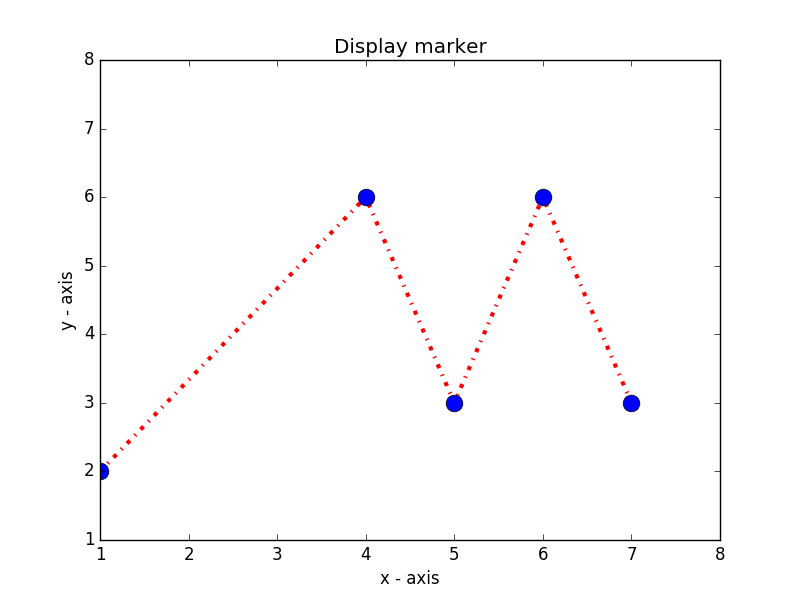﻿ Matplotlib Basic: Plot two or more lines and set the line markers - w3resource# Matplotlib Basic: Plot two or more lines and set the line markers

## Matplotlib Basic: Exercise-8 with Solution

Write a Python program to plot two or more lines and set the line markers.

Sample Solution:

Python Code:

``````import matplotlib.pyplot as plt
# x axis values
x = [1,4,5,6,7]
# y axis values
y = [2,6,3,6,3]

# plotting the points
plt.plot(x, y, color='red', linestyle='dashdot', linewidth = 3,
marker='o', markerfacecolor='blue', markersize=12)
#Set the y-limits of the current axes.
plt.ylim(1,8)
#Set the x-limits of the current axes.
plt.xlim(1,8)

# naming the x axis
plt.xlabel('x - axis')
# naming the y axis
plt.ylabel('y - axis')

# giving a title to my graph
plt.title('Display marker')
# function to show the plot
plt.show()
```
```

Sample Output:Python Code Editor: